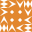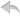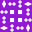注册    登录创作新主题

Python
 python开源   Django   Python   DjangoApp   pycharm
DATA
 docker   Elasticsearch

 问与答   闲聊   招聘   翻译   创业   分享发现   分享创造   求职   区块链   支付之战
WEB开发
 linux   MongoDB   Redis   DATABASE   NGINX   其他Web框架   web工具   zookeeper   tornado   NoSql   Bootstrap   js   peewee   Git   bottle   IE   MQ   Jquery

Python88.com
 反馈   公告   社区推广

Python社区  »  Python

# 我需要用python从范围数中的一个减去另一个得到结果

Yevi22 • 5 月前 • 64 次点击

1000-10=990比我需要的数字990-40=950以及循环到500的所有值都要多。我需要有代码来做这件事。

``````1000, 990
950, 940
900, 890
850, 840
800, 790
750, 740
700, 690
650, 640
600, 590
550, 540
``````

``````import math
x = 1000
y = 10
z = x-y
d = 40
print(x, z, sep=', ')
``````

``````for x in range(1000, 500, -40):
print(x)
``````

``````1000
960
920
880
840
800
760
720
680
640
600
560
520
``````

Python社区是高质量的Python/Django开发社区•   1 楼 desmond_jones    5 月前 您可以使用生成器函数。 ``````def generate_numbers(start, end): n = start yield n while True: for step in (10, 40): n -= step if n < end: return yield n `````` 用法: ``````for num in generate_numbers(1000, 500): print(num) `````` 要列出值,请使用 ``` list ``` 上课。 ``````list_nums = list(generate_numbers(1000, 50)) ``````•   2 楼 user9730851    5 月前 ``````n = 1000 while n > 500: print(n, end=', ') n -= 10 print(n) n -= 40 ``````